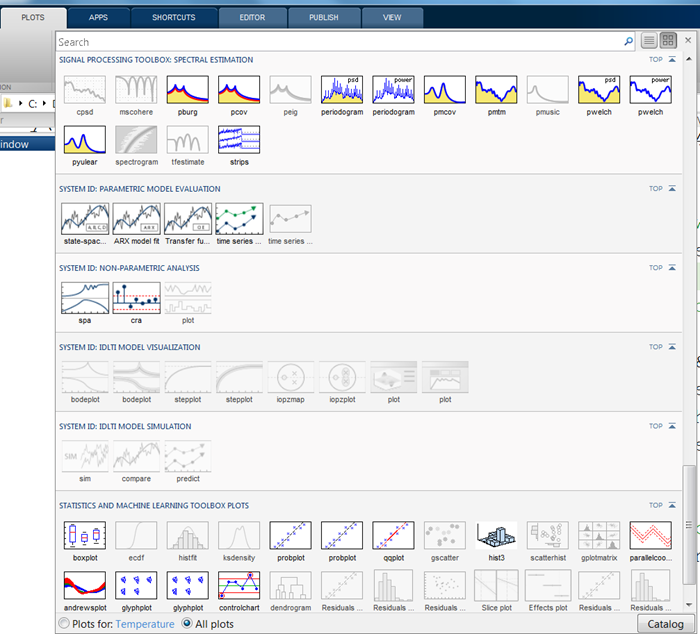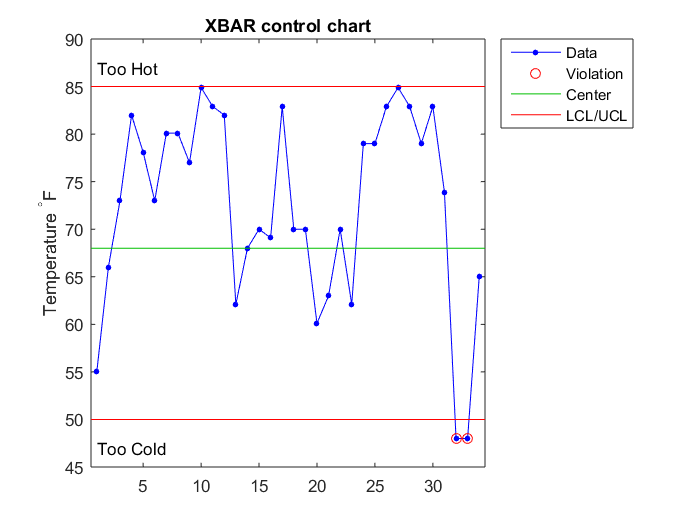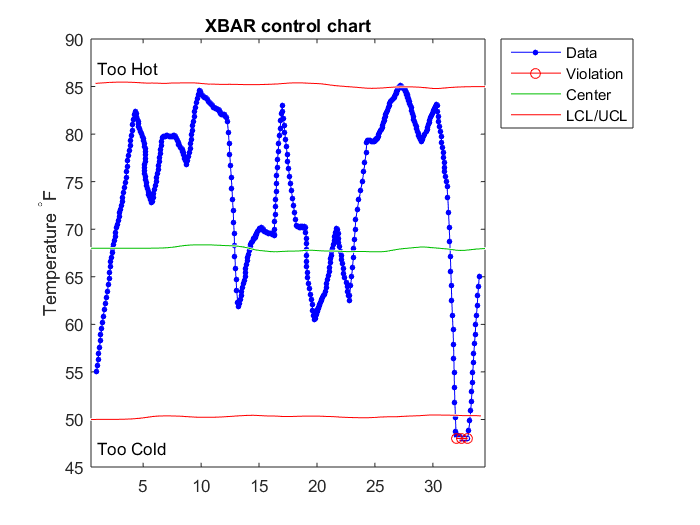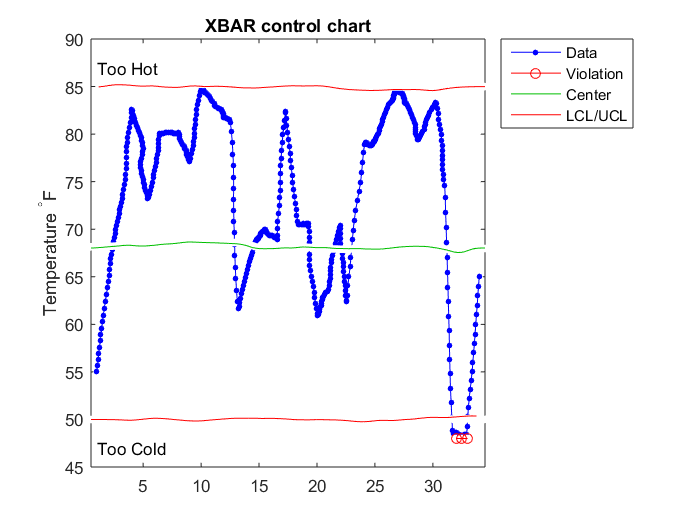# XKCDIFY

Sean's pick this week is xkcdify by Stuart Layton.

MATLAB has lots of stock plotting routines and many more that come with the various toolboxes. You can see the ones available to you on the plots tab:However, all of these plots are very formal. MATLAB doesn't have any stock options for informal plotting. The comic strip xkcd does a very good job communicating data in an informal way and Stuart has given us xkcdify to take our existing MATLAB plots and xkcdify or informalize them.

We've had some unseasonably cold weather in Massachusetts over the last few days (surprise I know!). Let's take a look at it with a control chart before and after xkcdification. I'll use the temperatures that I'm comfortable in a t-shirt and shorts for as the limits.

Before

controlchart(Temperature,'limit',[50 68 85])
ylabel('Temperature ^\circF')
ylim([45 90])
text(1,47,'Too Cold')
text(1,87,'Too Hot')After

xkcdify(gca)If this isn't informal enough, we can re-xkcdify too!

xkcdify(gca)There are a couple changes I made to xkcdify to make it work on more plot types.

• To separate a line into multiple pieces, you can use NaN. For the length of line calculation, I removed these NaNs because otherwise the line length was always NaN.
% Line 209:210
goodIdx = ~isnan(x) & ~isnan(y) & isfinite(x) & isfinite(y);
len = sum(hypot(diff(x(goodIdx)),diff(y(goodIdx))));
• There are cases where a line can be empty so I put an isempty() check around this.
% Line 129:132
if ~isempty(x)
x = x + smooth( generateNoise(n) .* rand(n,1) .* jx )';
y = y + smooth( generateNoise(n) .* rand(n,1) .* jy )';
end